We wrote two other blogs on this topic but pursued Harry Zumbrun to write something when those two other blogs were not what one of our customers wanted.

Those other blogs can be found here:

1. How to Convert Force to Mass http://www.mhforce.com/BlogPost/PostDetails/205?title=How-to-Convert-Force-to-Mass

2. Force Applications for Mass Reference Standard  http://www.mhforce.com/BlogPost/PostDetails/180?title=Force-Applications-for-Mass-Reference-Standards-

ADJUSTING LBF (POUNDS FORCE) WEIGHTS FOR GRAVITY AT POINT OF USE

When weights are used to perform force calibrations they should be corrected for the gravity and air buoyancy at the point of use.

Where the weights values are given in true mass the force in lbf is determined using the following formula: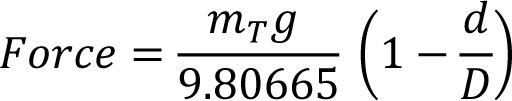Where the weight values are given in conventional mass the force value in lbf is determined using the following formula: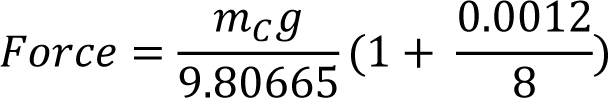When manufacturing and adjusting force weights in lbf it is desirable to adjust them to a nominal force value.  To determine the true mass value required to apply a given force use the following formula: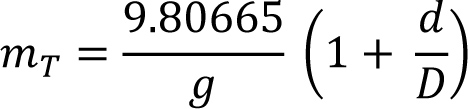Where:

mT = true mass of the weight

mC = conventional mass of the weight

g = local acceleration due to gravity

d = local air density (usually around 0.0012 Mg/m3)

D = density of the weight in the same units as d

Lastly, sometimes force weights adjusted in lbf for a specific gravity to be used at another location with a different gravity but the same air density.  This requires a correction factor be calculated as follows:

Force weights adjusted for use at gravity value:  980665 mgals

To be used at location with gravity value:  979563.577  mgals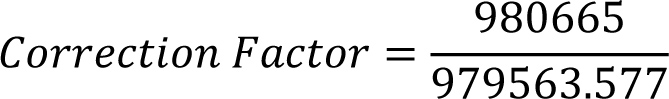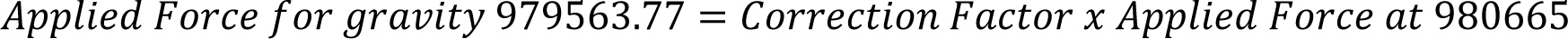This value used only if working in pounds-force; highly recommend performing all calculations in SI units and true mass with conversion to other unit systems at the end if needed

All parties must understand what this means and why conventional is being used (old vernacular used apparent mass versus brass in normal air with density of 8.4 for the brass, same air density as shown)

Tate (N.I.S.T. Technician) used this method 50 years ago; again, use of 9.80665 indicates pounds-force which means the true mass is in pounds

It works, however, note that no air buoyancy correction is shown.  If air buoyancy is to be considered, might be best to use the original equation and plug in the required new values for local gravitational acceleration and local air buoyancy

written by Harry Zumbrun

www.mhforce.com